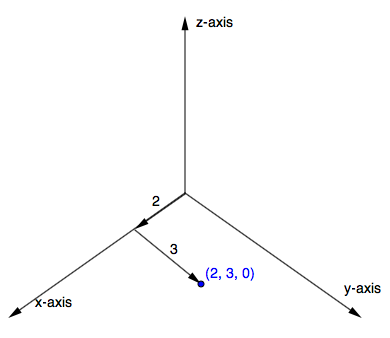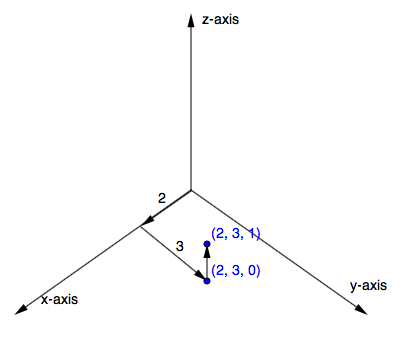### Home > PC > Chapter 10 > Lesson 10.2.1 > Problem10-68

10-68.

Have you ever thought of how to graph in three dimensions? The $x$- and $y$-axes are the same as usual and we generally think of them as lying in a horizontal plane. The $z$-axis goes through the origin and is perpendicular to the plane. (We generally think of it as being vertical.)

To get ready for graphing parametric equations in three dimensions, complete the following problems.

1. Draw the $x$- and $y$- axes in the plane.

2. On your paper, mark the points with coordinates $\left(2, 3\right)$ and $\left(5, 0\right)$.

3. Now imagine the $z$-axis going up from the origin. Why is the point with the 3-dimensional coordinate $\left(2, 3, 0\right)$ one of those you have already marked?4. Place the tip of your pencil at the location where the points $\left(2, 3, 1\right)$, $\left(2, 3, 5\right)$, $\left(5, 0, 2\right)$, and $\left(5, 0, −1\right)$ would be.5. One of the points in part (d) was below the paper. Which one?